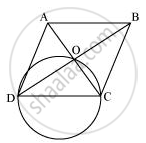# Prove that the Circle Drawn with Any Side of a Rhombus as Diameter Passes Through the Point of Intersection of Its Diagonals. - Mathematics

Prove that the circle drawn with any side of a rhombus as diameter passes through the point of intersection of its diagonals.

#### SolutionLet ABCD be a rhombus in which diagonals are intersecting at point O and a circle is drawn while taking side CD as its diameter. We know that a diameter subtends 90° on the arc.

∴ ∠COD = 90°

Also, in rhombus, the diagonals intersect each other at 90°.

∠AOB = ∠BOC = ∠COD = ∠DOA = 90°

Clearly, point O has to lie on the circle.

Is there an error in this question or solution?
Chapter 10: Circles - Exercise 10.6 [Page 186]

#### APPEARS IN

NCERT Class 9 Maths
Chapter 10 Circles
Exercise 10.6 | Q 5 | Page 186

Share